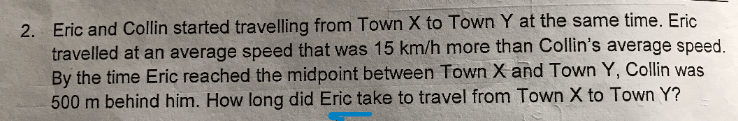# Questionneed some help on the speed question.

appreciate any tips given

thanks

500 m = 0.5 km
0.5/15 = 1/30 h (time taken for Eric to reach the midpoint)
(1/30) x 2 = 1/15 h = 4 min

Ans : 4 min.

how do we conclude the distance from home to midpoint is 500m?

The distance from home to midpoint is not 500 m. 500 m was the additional distance that Eric had travelled due to the faster speed of ’15 km/h more’. Distance travelled/average speed = time taken (to reach the midpoint)